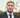# PHP Weighted Random Choice & Selection Remove / Replacement

October 20, 2010

For something I have been doing in a php project of late, I needed to weight an array of values and as I go round and select the values remove the value or -1 from the weight depending on how many of the value are left in the weight.

If you have any improvements or suggestions then id very much like to here them :-)

```\$starttime = microtime();
\$startarray = explode(” ”, \$starttime);
\$starttime = \$startarray + \$startarray;
/**

weighted_random()
Pick a random item based on weights.
*
@param array \$values Array of elements to choose from
@param array \$weights An array of weights. Weight must be a positive number.

@return mixed Selected element.
*/
function weightedrandom(\$values, \$weights){
\$count = count(\$values);
\$i = 0;
\$n = 0;
\$num = mtrand(0, array_sum(\$weights));
while(\$i < \$count){
\$n += \$weights[\$i];
if(\$n >= \$num){
break;
}
\$i++;
}
return \$i;
}
function recalc(\$val,\$values,\$weight){
```//two steps to consider here, -1 from the weight and if the weight is &lt;=0 unset both the value and the weight.
if(\$weight[\$val]-1&lt;=0){
unset(\$weight[\$val]);
unset(\$values[\$val]);

\$new_values = array();
\$new_weight = array();

foreach(\$values as \$k=&gt;\$v){
\$new_values[]=\$v;
\$new_weight[]=\$weight[\$k];
}

return array(\$new_values,\$new_weight);

}else{
\$weight[\$val]=\$weight[\$val]-1;
return array(\$values,\$weight);
}```
}
//in the context of what im doing lets get this right.
\$values = array(0=>“164-2”,1=>“164-1”,2=>“2-2”);
\$weights = array(0=>1,1=>2,2=>1);
//now we wanna select 4 values but edit them as we go round.
\$find = 3;
for(\$i=0;\$i<\$find;\$i++){
```\$val = weighted_random(\$values,\$weights);
echo \$val.'  |  ';
echo \$values[\$val].'<br/>';

list(\$values,\$weights) = recalc(\$val,\$values,\$weights);```
}
echo ‘The new weights are ’;
print_r(\$weights);
echo ’‘;
\$endtime = microtime();
\$endarray = explode(” ”, \$endtime);
\$endtime = \$endarray + \$endarray;
\$totaltime = \$endtime - \$starttime;
\$totaltime = \$totaltime;